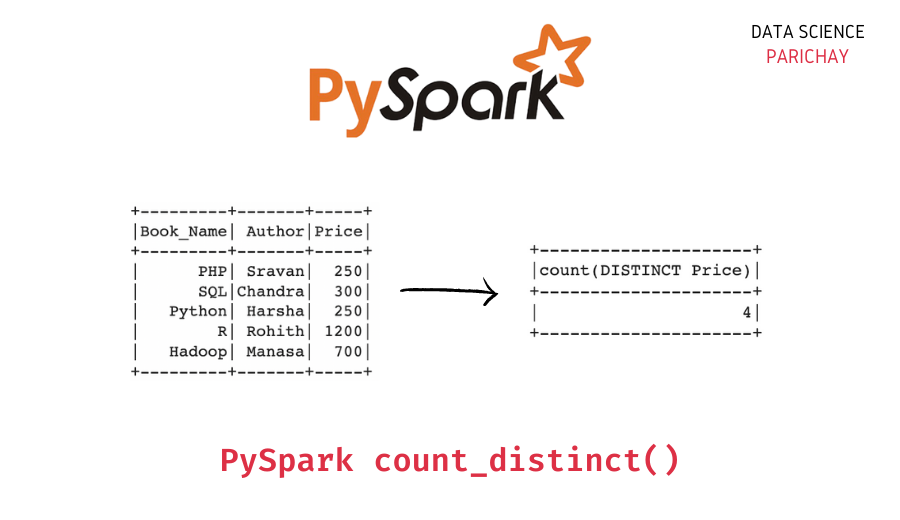# Pyspark – Count Distinct Values in a Column

In this tutorial, we will look at how to get a count of the distinct values in a column of a Pyspark dataframe with the help of examples.

## How to count unique values in a Pyspark dataframe column?

You can use the Pyspark `count_distinct()` function to get a count of the distinct values in a column of a Pyspark dataframe. Pass the column name as an argument. The following is the syntax –

`count_distinct("column")`

It returns the total distinct value count for the column.

## Examples

Let’s look at some examples of getting the count of unique values in a Pyspark dataframe column. First, let’s create a Pyspark dataframe that we’ll be using throughout this tutorial.

```#import the pyspark module
import pyspark

# import the  sparksession class  from pyspark.sql
from pyspark.sql import SparkSession

# create an app from SparkSession class
spark = SparkSession.builder.appName('datascience_parichay').getOrCreate()

# books data as list of lists
df = [[1, "PHP", "Sravan", 250],
[2, "SQL", "Chandra", 300],
[3, "Python", "Harsha", 250],
[4, "R", "Rohith", 1200],
]

# creating dataframe from books data
dataframe = spark.createDataFrame(df, ['Book_Id', 'Book_Name', 'Author', 'Price'])

# display the dataframe
dataframe.show()```

Output:

```+-------+---------+-------+-----+
|Book_Id|Book_Name| Author|Price|
+-------+---------+-------+-----+
|      1|      PHP| Sravan|  250|
|      2|      SQL|Chandra|  300|
|      3|   Python| Harsha|  250|
|      4|        R| Rohith| 1200|
+-------+---------+-------+-----+```

We now have a dataframe with 5 rows and 4 columns containing information on some books.

### Count distinct values in a column

Let’s count the distinct values in the “Price” column. For this, use the following steps –

1. Import the `count_distinct()` function from `pyspark.sql.functions`.
2. Use the `count_distinct()` function along with the Pyspark dataframe `select()` function to count the unique values in the given column.
```# import count_distinct function
from pyspark.sql.functions import count_distinct

# distinct value count in the Price column
dataframe.select(count_distinct("Price")).show()```

Output:

📚 Data Science Programs By Skill Level

Introductory

Intermediate ⭐⭐⭐

🔎 Find Data Science Programs 👨‍💻 111,889 already enrolled

Disclaimer: Data Science Parichay is reader supported. When you purchase a course through a link on this site, we may earn a small commission at no additional cost to you. Earned commissions help support this website and its team of writers.

```+---------------------+
|count(DISTINCT Price)|
+---------------------+
|                    4|
+---------------------+```

We find that the “Price” column has 4 distinct values. If you see the dataframe above, you can see that two books have the same price of 250, and the other three books have different prices.

### Count distinct values in multiple columns in Pyspark

You can also get the distinct value count for multiple columns in a Pyspark dataframe. Let’s count the unique values in the “Author” and the “Price” columns of the above dataframe.

```# import count_distinct function
from pyspark.sql.functions import count_distinct

# distinct value count in the Author and the Price columns
dataframe.select(count_distinct("Author"), count_distinct("Price")).show()```

Output:

```+----------------------+---------------------+
|count(DISTINCT Author)|count(DISTINCT Price)|
+----------------------+---------------------+
|                     5|                    4|
+----------------------+---------------------+```

Here, we use a `count_distinct()` function for each column we want to compute the distinct count of inside the `select()` function. You can see that the “Author” column has 5 distinct values whereas the “Price” column has 4 distinct values.

You might also be interested in –

••